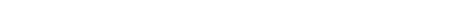### 11.3 Graphics state

The graphics-state object associated with each port holds values for parameters as shown below.

Note: the graphics state parameters stipple , pattern , fill-style , mask-x and mask-y are supported only on Unix.

###### Parameters held in a graphics-state objectParameter Name

Default Value

Allowed Values

transform

the unit transform

Anything returned by the transform functions. (See below)

foreground

``` :black```

A color spec, pixel, or symbol

background

``` :white```

A color spec, pixel, or symbol

operation

``` boole-1```

Boole constants (Chapter 12 of the ANSI standard)

stipple

``` nil```

A bitmap or ``` nil```

pattern

``` nil```

A pixmap of same depth as port, or ``` nil```

fill-style

``` :solid```

``` :solid, :opaque-stippled, :stippled``` or ``` :tiled```

thickness

``` 1```

``` number```

scale-thickness

``` t```

``` nil``` or ``` t```

dashed

``` nil```

``` nil``` or ``` t```

dash

'``` (4 4)```

A list of two or more integers

line-end-style

``` :butt```

``` :butt``` , ``` :round``` or ``` :projecting```

line-joint-style

``` :miter```

``` :bevel``` , ``` :miter``` or ``` :round```

mask

``` nil```

``` nil``` or a list of the form
``` (``` x y width height ``` )```

mask-x

``` 0```

``` integer```

mask-y

###### ``` 0```

``` integer```

font

###### ``` nil```

``` nil``` or a valid font

transform

An object which determines the coordinate transformation applying to the graphics port. The default value leaves the port coordinates unchanged from those used by the host window system -- origin at top left, X increasing to the right and Y increasing down the screen. Graphics state transforms describes these objects.

foreground

Determines the foreground color used in drawing functions. A color can be a pixel value, a color name symbol, a color name string or a color object. Using pixel values results in better performance.

background

Determines the background color used in drawing functions which use a stipple. Valid values are the same as for foreground .

operation

Determines the color combination used in the drawing primitives. Valid values are 0 to 15, being the same logical values as the op arg to the Common Lisp function ``` boole``` . Graphics state operation shows how to use operation .

Note: Graphics State operation is not supported by Cocoa/Core Graphics so this slot or argument is ignored on Cocoa.

stipple

A 1-bit pixmap ("bitmap") or ``` nil``` . The bitmap is used in conjunction with the ``` fill-style``` when drawing. Here, ``` nil``` means that all pixels are drawn in the ``` foreground``` color. A stipple is not transformed by the ``` transform``` parameter. Its origin is assumed to coincide with the port's. The ``` stipple``` is tiled across the drawing. ``` stipple``` is ignored if a ``` pattern``` is given. If no ``` fill-style``` is given, or it is specified as ``` :solid``` , when a ``` Stipple``` is given, then ``` fill-style``` defaults to ``` :opaque-stippled``` .

fill-style

One of ``` :solid``` ``` :opaque-stippled``` or ``` :stippled``` . Its value determines how the drawing is done. The default value ``` :solid``` means that the ``` foreground``` is used everywhere. ``` :opaque-stippled``` means that the ``` stipple``` bitmap is used with stipple 1s giving the ``` foreground``` and 0s the ``` background``` . ``` :stippled``` means that the ``` stipple``` bitmap is used with ``` foreground``` where there are 1s and where the are 0s, no drawing is done. If you specify a stipple but no ``` fill-style``` , or a ``` fill-style``` of ``` :solid``` , it defaults to ``` :opaque-stipple``` .

pattern

A pixmap the same depth as the port. It is used as the source of color for drawing instead of the ``` foreground``` and ``` background``` parameters. A pattern is not transformed by the ``` transform``` parameter. The ``` pattern``` is tiled across the drawing. When ``` pattern``` is specified, the ``` stipple``` value is ignored.

thickness

The thickness of lines drawn. If ``` scale-thickness``` is non-``` nil``` , the value is in port (transformed) coordinates, otherwise it is in pixels.

scale-thickness

If non-``` nil``` means interpret the ``` thickness``` parameter in transformed port coordinates, otherwise interpret it in pixels.

dashed

If non-``` nil``` draws a dashed line using dash as the mark-space specifier.

dash

If non-``` nil``` should be a list of integers specifying the alternate mark and space sizes for dashed lines. The mark and space values are interpreted in pixels only.

line-end-style

One of ``` :butt``` ``` :round``` or ``` :projecting``` and specifies how to draw the ends of lines.

line-joint-style

One of ``` :bevel``` ``` :miter``` ``` :round``` and specifies how to draw the areas where the edges of polygons meet.

mask

Either ``` nil``` or a list of the form ``` (``` x y width height ``` )``` , defining a rectangle inside which the drawing is done. The ``` mask``` is not tiled. A mask is not transformed by the ``` transform``` parameter.

mask-x

An integer specifying where in the port the X coordinate of the mask origin is to be considered to be. The value is in window coordinates.

mask-y

An integer specifying where in the port the Y coordinate of the mask origin is to be considered to be. The value is in window coordinates.

font

Either ``` nil``` or a valid font name or font object to be used by the ``` draw-character``` and ``` draw-string``` functions. A valid font is a portable font description. See Portable font descriptions.

#### 11.3.1 Setting the graphics state

LispWorks CAPI User Guide (Macintosh version) - 8 Apr 2005